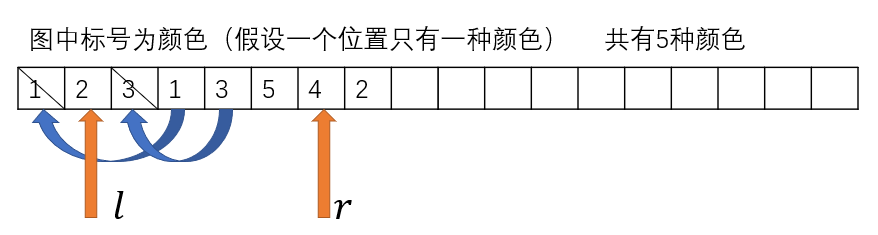# 洛谷 P2564 [SCOI2009]生日礼物 题解【贪心】【堆】【线段树】【two-pointer】

是一个贪心，不过解法比较多。

## 输入输出样例

6 3
1 5
2 1 7
3 1 3 8


3

【样例说明】

【数据规模】

## 题解：

这个题可以先排序，从左到右做，每次更新这个颜色最后一次出现的位置，放入线段树。如果所有颜色都出现过，就去线段树中查询所有颜色最后一次出现时间的最小值。每次更新当前位置与这个最小值之差的最小值作为答案，时间复杂度为$O(N(\log n+\log k))$。线段树长度只用开$k$即可。

正解是类似two-pointer的尺取法，也就是先排序，从左到右找，定义一个左端点初始化为0。每次找到一个新的颜色就把前面的颜色打上删除标记，等找到全部颜色以后就从左端点开始枚举，把左端点调整到第一个没有删除标记的位置，这就是到当前位置的最优答案。当我们第一次发现所有的颜色，是在第7个位置，此时的左端点为1，我们从左边开始检索，找到第一个没有被删除的位置：2。那么我们找到的第一个答案就是7-2=5，更新到最小值中去。再继续遍历，发现2，此时把左边的2删除，这时左端点可以被移到4号位置去，更新最小值就是8-4=4。时间复杂度是$O(N\log N+K)$

不过这个题的数据规模是$10^6$，跑$O(N\log N)$的排序有的评测机就可能吃不消。我们其实还可以优化。

注意到题目给出的位置都是升序，那联想到归并排序合并的过程，我们只要每次找到$k$列中剩下的最小的元素取出来不就可以了吗。在$k$列中找最小只需要一个$k$大小的堆就可以了。这样排序，对于很小很小的$k$来说，近似$O(N)$。时间复杂度为$O(N\log K+K)$。

## Code of two-pointer：

#include<cstdio>
#include<cstring>
#include<vector>
using std::vector;//因为不知道每一种颜色有多少个所以用vector
struct node
{
int c,v;
node(int c,int v)
{
this->c=c;
this->v=v;
}
node()
{}
friend bool operator <(node a,node b)
{return a.v<b.v;}

friend bool operator >(node a,node b)
{return a.v>b.v;}

}h,b;
int l=0;
//手写堆部分，优化常数
void push(node k)
{
h[++l]=k;
int i=l;
while(i>1&&h[i]<h[i>>1])
{
node t=h[i];
h[i]=h[i>>1];
h[i>>1]=t;
i>>=1;
}
return;
}
void pop()
{
int i=1;
h=h[l--];
while((i<<1)<=l&&(h[i]>h[i<<1]||h[i]>h[i<<1|1]))
if((i<<1)==l||h[i<<1]<h[i<<1|1])
{
node t=h[i];
h[i]=h[i<<1];
h[i<<1]=t;
i<<=1;
}
else
{
node t=h[i];
h[i]=h[i<<1|1];
h[i<<1|1]=t;
i=i<<1|1;
}
return;
}
vector<int> v;
int tt,now;//tt是总共的个数，now是现在的个数，用于堆排序
int lst;//某种颜色上一次出现的位置
int used;//颜色出现过没有，used表示一共出现了多少种颜色
int del,st=1;//删除标记和左端点
int main()
{
memset(lst,0,sizeof(lst));
int n,k,u;
scanf("%d%d",&n,&k);
for(int i=1;i<=k;i++)
now[i]=1;
for(int i=1;i<=k;i++)
{
scanf("%d",&tt[i]);
for(int j=1;j<=tt[i];j++)
{
scanf("%d",&u);
v[i].push_back(u);
}
}

for(int i=1;i<=k;i++)
push(node(i,v[i]));
for(int i=1;i<=n;i++)//排序过程
{
b[i]=h;
pop();
if(now[b[i].c]<tt[b[i].c])
push(node(b[i].c,v[b[i].c][now[b[i].c]]));
now[b[i].c]++;
}
int ans=0x7fffffff;
for(int i=1;i<=n;i++)
{
if(!used[b[i].c])
{
used[b[i].c]=1;
used++;
}
del[lst[b[i].c]]=true;
lst[b[i].c]=i;
if(used==k)
{
while(del[st])//删除该删除的
st++;
ans=ans<(b[i].v-b[st].v)?ans:(b[i].v-b[st].v);//更新答案
}
}
printf("%d\n",ans);
return 0;
}


## Code of Segment tree：

#include<cstdio>
#include<cstring>
#include<algorithm>
#define ls (k<<1)
#define rs (k<<1|1)
#define mid (l+r>>1)
#define Mid (t[k].l+t[k].r>>1)
int Min(int x,int y)
{
return x<y?x:y;
}
struct node
{
int l,r,v;
node(int l,int r)
{
this->l=l;
this->r=r;
v=-1;
}
node()
{
v=-1;
}
}t;
void build(int k,int l,int r)
{
t[k]=node(l,r);
if(l==r)
return;
build(ls,l,mid);
build(rs,mid+1,r);
}
void change(int k,int p,int x)
{
if(t[k].l==t[k].r)
{
t[k].v=x;
return;
}
if(p<=Mid)
change(ls,p,x);
else
change(rs,p,x);
t[k].v=Min(t[ls].v,t[rs].v);
return;
}
{
if(t[k].l==l&&t[k].r==r)
return t[k].v;
if(r<=Mid)
else if(l>Mid)
else
}

struct ball
{
int c,p;
friend bool operator <(ball a,ball b)
{
return a.p<b.p;
}
}a;
int cnt=0;
int main()
{
int n,k,t,u;
scanf("%d%d",&n,&k);
build(1,1,k);
for(int i=1;i<=k;i++)
{
scanf("%d",&t);
for(int j=1;j<=t;j++)
{
scanf("%d",&u);
a[++cnt].c=i;
a[cnt].p=u;
}
}
std::sort(a+1,a+1+n);
int ans=0x7fffffff;
for(int i=1;i<=n;i++)
{
change(1,a[i].c,a[i].p);
if(tmp==-1)//为-1说明还有颜色没找到
continue;
ans=ans<(a[i].p-tmp)?ans:(a[i].p-tmp);
}
printf("%d\n",ans);
return 0;
}


### 说点什么Subscribe

/* */